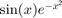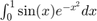# Homework #3

All problems must be in a single *.m file. You will need an additional file for a function. Make a separate block in the code for each problem. Do not submit individual files for each problem. See previous solutions.

## Problem 1 (3 points)

Create a function that will perform a numerical integration using either the midpoint, trapezoid, or Simpson's rule method. The inputs to the function should be (in this order): function handle to function being integrated, Lower Limit, Upper Limit, Integration type. Integration type is 'MidPoint', 'Trapezoid', or 'Simpson'. Example:

[Out] = LastName_HW3_myIntFunc(FuncHandle, A,B, 'MidPoint')

Create an anonymous function forFind the integralusing 20 intervals. An interval is defined as 'a' to 'b' as seen here: https://en.wikipedia.org/wiki/Simpson's_rule

Calculate and show the result using each method.

## Problem 2 (2 points)

Create a 5x5 'magic' matrix ('help magic').

Verify that the sum along all rows and all columns is the same.

Use MATLAB to find how many elements are larger than 10.

Use indexing by a logical matrix to replace all elements larger than 10 by the value 555